# How to Calculate and Solve for Degree of Polymerization | Polymer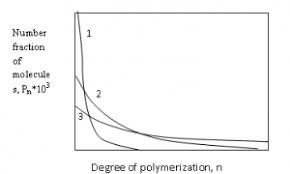The image of degree of polymerization is represented above.

To compute for degree of polymerization, two essential parameters are needed and these parameters are Mean Number Molecular Weight (M’nand Unit Molecular Weight (m).

The formula for calculating degree of polymerization:

D.P. = M’n/m

Where:

D.P. = Degree of Polymerization
M’n = Mean Number Molecular Weight
m = Unit Molecular Weight

Let’s solve an example;
Find the degree of polymerization when the mean number molecular weight is 28 and the unit molecular weight is 4.

This implies that;

M’n = Mean Number Molecular Weight = 28
m = Unit Molecular Weight = 4

D.P. = 28/4
D.P. = 7

Therefore, the degree of polymerization is 7.

Calculating the Mean Number Molecular Weight when the Degree of Polymerization and the Unit Molecular Weight is Given.

M’n = D.P (m)

Where;

M’n = Mean Number Molecular Weight
D.P. = Degree of Polymerization
m = Unit Molecular Weight

Let’s solve an example;
Find the mean number molecular weight when the degree of polymerization is 6 and the unit molecular weight is 4.

This implies that;

D.P. = Degree of Polymerization = 6
m = Unit Molecular Weight = 4

M’n = D.P (m)
M’n = 6 (4)
M’n = 24

Therefore, the degree of polymerization is 24.

Calculating the Unit Molecular Weight when the Degree of Polymerization and the Mean Number Molecular Weight is Given.

m = M’n / D.P

Where:

m = Unit Molecular Weight
D.P. = Degree of Polymerization
M’n = Mean Number Molecular Weight

Let’s solve an example;
Given that the degree of polymerization is 10 and the mean number molecular weight is 40. Find the unit molecular weight?

This implies that;

D.P. = Degree of Polymerization = 10
M’n = Mean Number Molecular Weight = 40

m = M’n / D.P
m = 40 / 10
m = 4

Therefore, the degree of polymerization is 4.

Nickzom Calculator – The Calculator Encyclopedia is capable of calculating the degree of polymerization.

To get the answer and workings of the degree of polymerization using the Nickzom Calculator – The Calculator Encyclopedia. First, you need to obtain the app.

You can get this app via any of these means:

You can also try the demo version via https://www.nickzom.org/calculator

Apple (Paid) – https://itunes.apple.com/us/app/nickzom-calculator/id1331162702?mt=8
Once, you have obtained the calculator encyclopedia app, proceed to the Calculator Map, then click on Materials and Metallurgical under Engineering.Now, Click on Polymer under Materials and MetallurgicalNow, Click on Degree of Polymerization under Polymer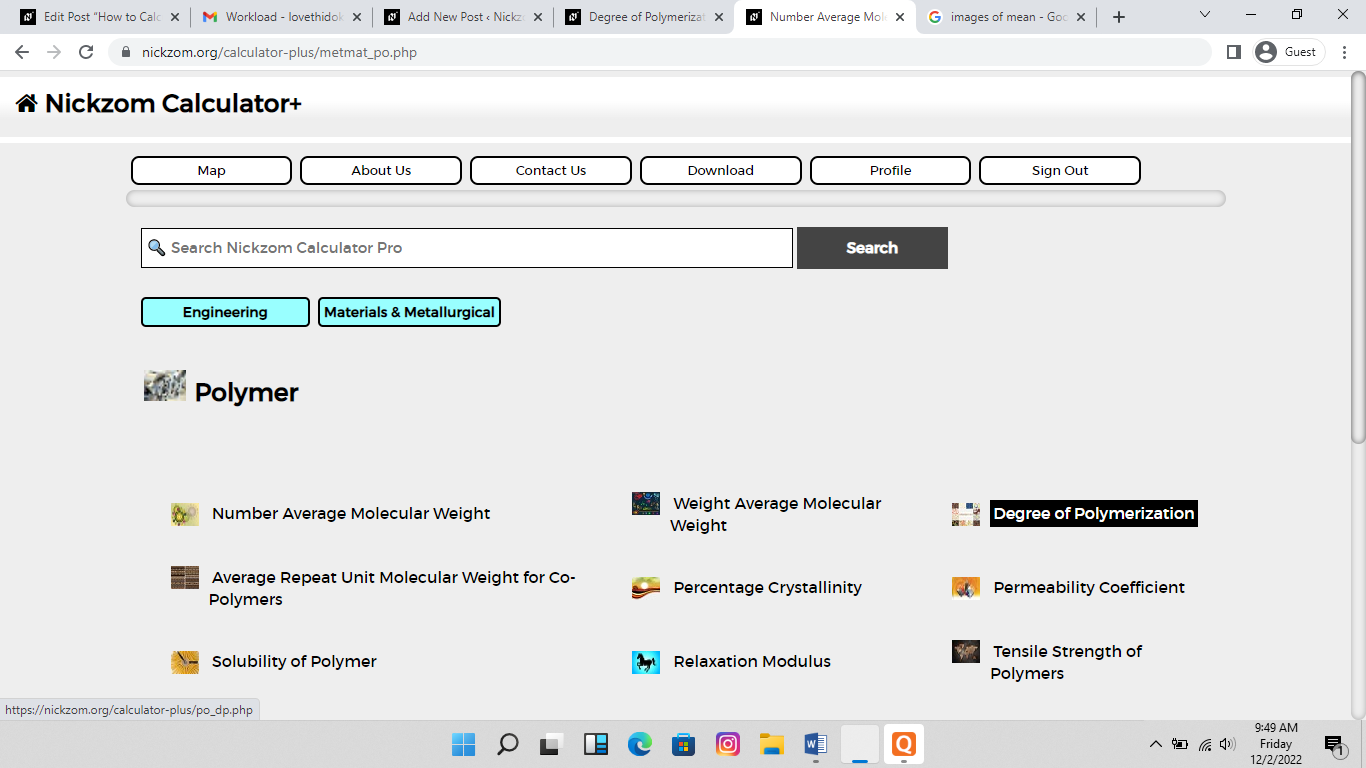The screenshot below displays the page or activity to enter your values, to get the answer for the degree of polymerization according to the respective parameter which is the Mean Number Molecular Weight (M’nand Unit Molecular Weight (m).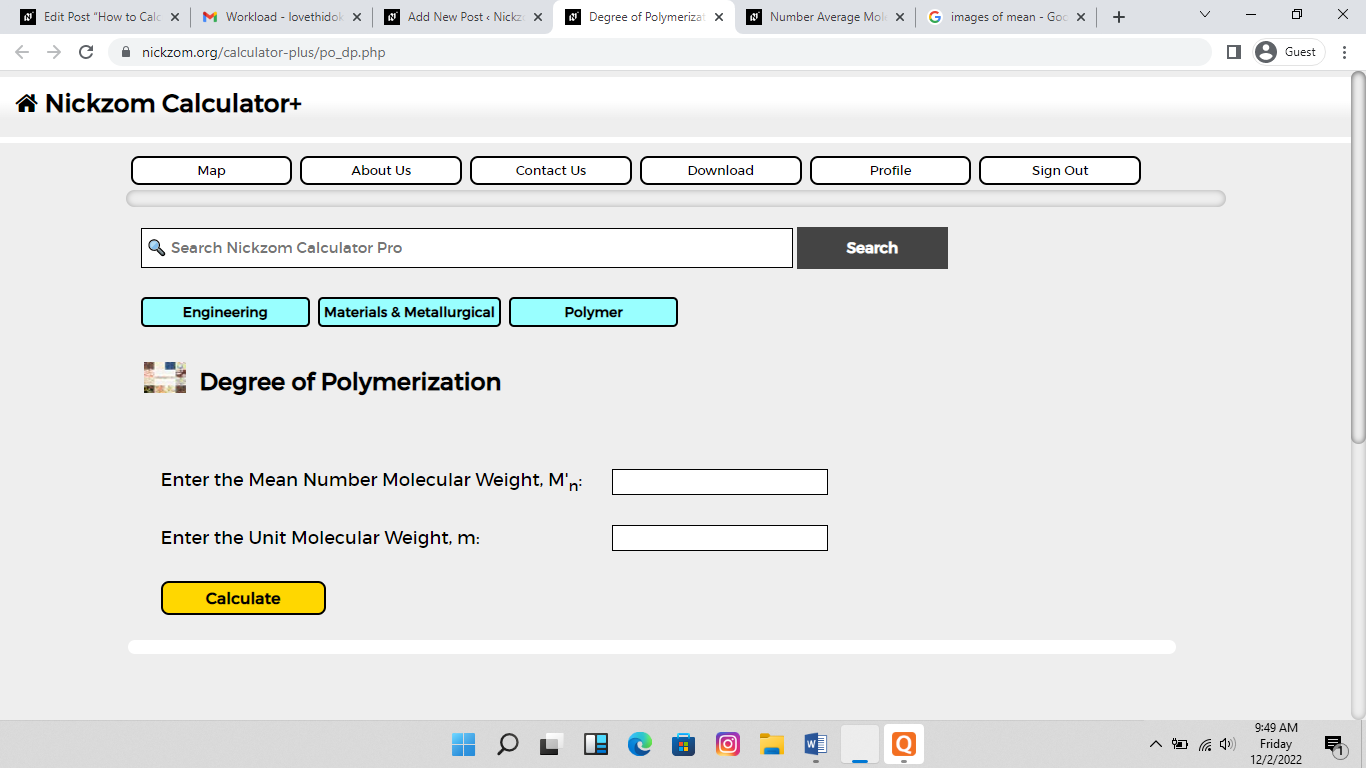Now, enter the values appropriately and accordingly for the parameters as required by the Mean Number Molecular Weight (M’n) is 28 and Unit Molecular Weight (m) is 4.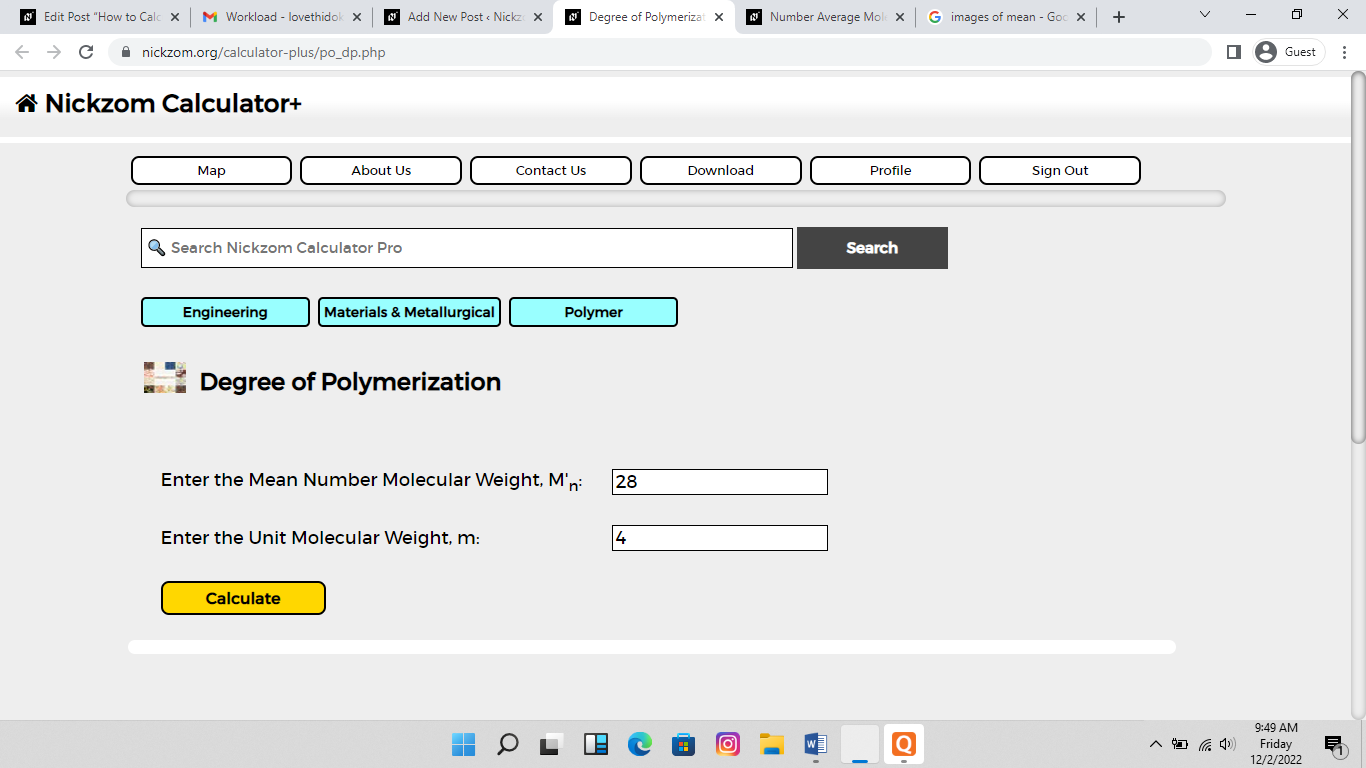Finally, Click on Calculate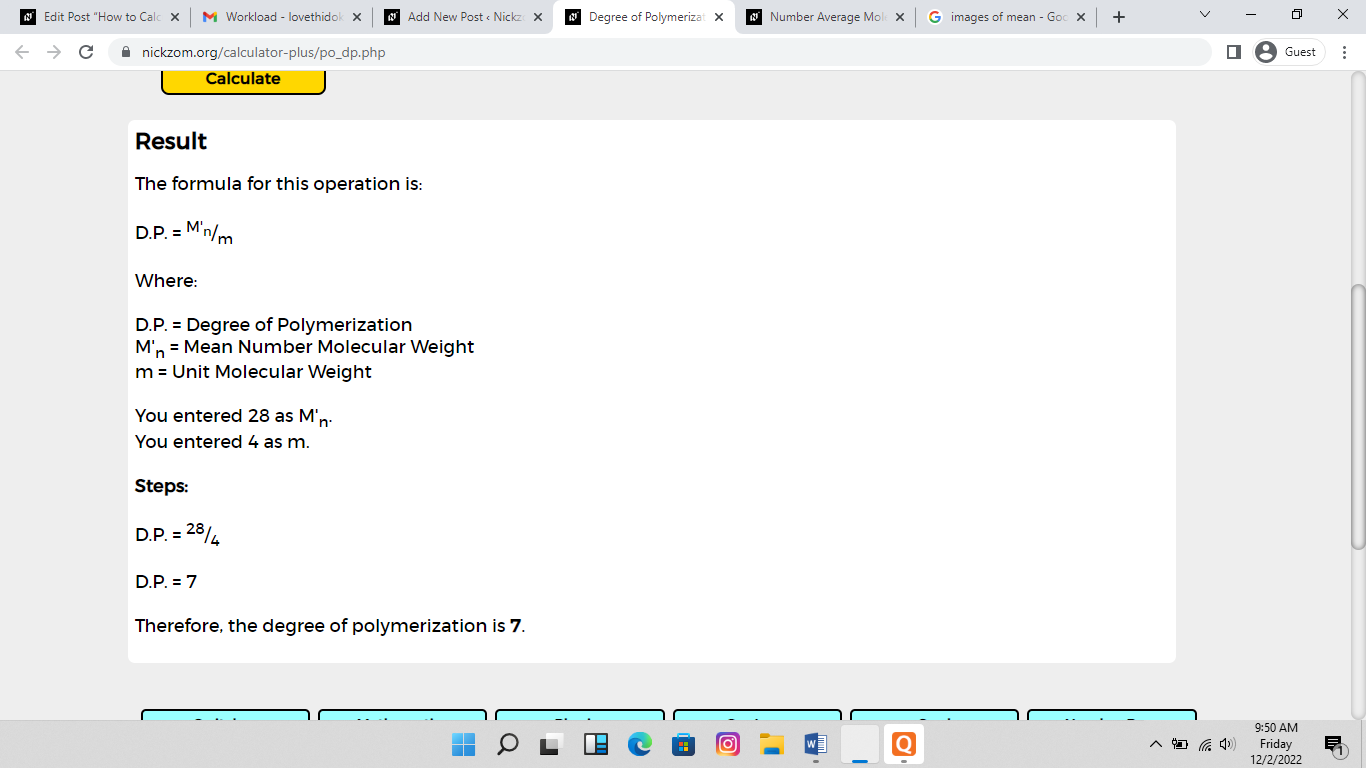As you can see from the screenshot above, Nickzom Calculator– The Calculator Encyclopedia solves for the degree of polymerization and presents the formula, workings and steps too.